# Altova StyleVision 2020 Enterprise Edition

 Example: An Invoice Scroll Home Prev Top Next More

The Invoice.sps example in the (My) Documents folder, C:\Documents and Settings\<username>\My Documents\Altova\StyleVision2020\StyleVisionExamples\Tutorial\Auto-Calculations\, demonstrates how Auto-Calculations can be used for the following purposes:

Counting nodes

Selecting a node based on input from the Authentic View user

Updating the content of a node with the result  of an Auto-Calculation

Using the result of one Auto-Calculation in another Auto-Calculation

In the example file, the Auto-Calculations have been highlighted with a yellow background color (see screenshot below).

## Counting nodes

In the Invoice example, each product in the list is numbered according to its position in the list of products that a customer has ordered (Product 1, Product 2, etc). This numbering is achieved with an Auto-Calculation (screenshot below).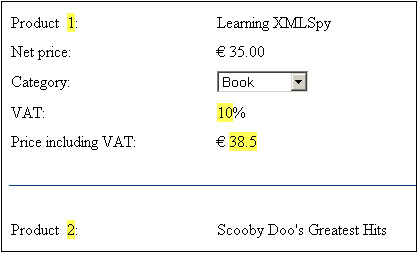In this particular case, the XPath expression position() would suffice to obtain the correct numbering. Another useful way to obtain the position of a node is to count the number of preceding siblings and add one. The XPath expression would be: count(preceding-sibling::Product)+1. The latter approach could prove useful in contexts where using the position() function is difficult to use or cannot be used. You can test this Auto-Calculation in the example file by deleting products, and/or adding and deleting new products.

## Selecting a node based on user input

In the Invoice example, the user selects the category of product (Book, CD, DVD, or Electronics) via a combo box. This selection is entered in the //Product/Category node in the XML document. An Auto-Calculation then uses this value to reference a "lookup table" in the XML document and identify the node holding the VAT percentage for this product category. The XPath expression of this Auto-Calculation is:

for \$i in Category return /Invoice/Categories/Category[. = \$i]/@rate.

The VAT percentage is displayed at the Auto-Calculation location in the output. In the Invoices example, the lookup table is stored in the same XML document as the invoice data. However, such a table can also be stored in a separate document, in which case it would be accessed using the doc() function of XPath 2.0. To test this Auto-Calculation, change the product type selection in any product's Category combo box; the VAT value will change accordingly (Book=10%; CD=15%; DVD=15%; Electronics=20%).

## Updating content of a node with the result of an Auto-Calculation

The VAT percentage obtained by the Auto-Calculation from the lookup is dynamic and stored temporarily in memory (for use in Authentic View). The result of the Auto-Calculation can, however, be stored in the VAT node in the XML document. This has two advantages: (i) the content of the VAT node does not have to be entered by the user; it is entered automatically by the Auto-Calculation; (ii) each time the lookup table is modified, the changes will be reflected in the VAT node when Invoice.xml is opened in Authentic. To cause an Auto-Calculation to update a node, select the Auto-Calculation in Design View, and in the Properties window (screenshot below), click the Authentic | Update XML Node button. In the dialog that pops up, select the VAT node, and then click OK.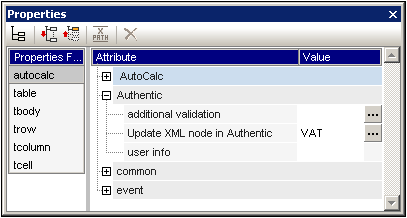In Authentic View, when the user selects a different product category in the combo box, the Auto-Calculation obtains the VAT percentage by referencing the lookup table, displays the VAT percentage, and updates the VAT node.

## Using an Auto-Calculation-updated node in another Auto-Calculation

The VAT percentage, obtained by the Auto-Calculation described above, is required to calculate the gross price (net price + VAT amount) of each product. The formula to use would be derived as follows:

Gross Price = Net Price + VAT-amount

Since VAT-amount = Net Price * VAT-percentage div 100

Gross Price = Net Price + (Net Price * VAT-percentage div 100)

The net price of a product is obtained from the PriceNet node. The VAT percentage is calculated by an Auto-Calculation as described above, and this Auto-Calculation updates the VAT node. The content of the VAT node can now be used in an Auto-Calculation to generate the gross price. The XPath expression to do this would be:

PriceNet  + (PriceNet * (VAT div 100))

The XPath expression can be viewed and edited in the Properties window. You can test the Auto-Calculation for the gross price by changing either the price or product category of any product. Notice that the gross price (price including VAT) of the product also changes.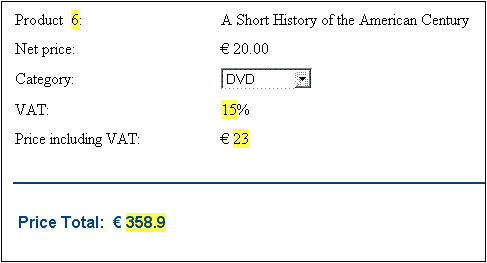In the Invoice SPS, the gross price Auto-Calculation updates the PriceGross node in the XML document.

The updated PriceGross nodes can now be used in an Auto-Calculation that sums up the prices of all purchased products. The XPath expression would be: sum(Order/Product/PriceGross). In the Invoice SPS, this Auto-Calculation updates the PriceTotal node. You can test this Auto-Calculation by modifying the prices of individual products and seeing the effect on the price total.

## An Auto-Calculation Exercise

Now add two Auto-Calculation components to the SPS yourself.

1.Create an Auto-Calculation that calculates a volume discount for the entire invoice. If the order amount (price total) exceeds Euro 100, Euro 300,  or Euro 600, discounts of 5%, 10%, and 12% apply, respectively. Display the discount amount (see screenshot below) and update the DiscountAmount node in the XML document.

2.Create an Auto-Calculation that calculates the discounted bill amount. This amount would be the price total less the discount amount (as calculated in the previous Auto-Calculation). Display the bill amount (see screenshot below) and update the BillAmount node in the XML document.

Set up these components so that the Authentic View output is as shown in the screenshot below.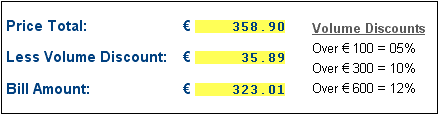You can see these two additional Auto-Calculations in the file InvoiceWithDiscounts.sps, which is in the (My) Documents folder, C:\Documents and Settings\<username>\My Documents\Altova\StyleVision2020\StyleVisionExamples\Tutorial\Auto-Calculations folder.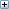See also